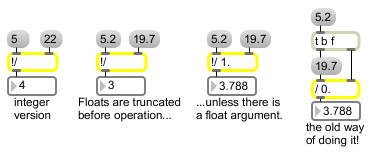!/

Division object (inlets reversed)

Description

The !/ object functions just like the / object, but the inlets' functions are reversed.

Arguments

Name Type Opt Description
initial-divisor int or float opt Sets an initial value for the divisor. If there is no argument, the divisor is set to 1 initially. A float argument causes the numbers to be divided as floats. (Division by 0 is not allowed. Int division by 0 will have the same result as dividing by 1. Float division by 0 will always cause an output of -2^31.)

Messages

 bang In left inlet: Performs the division with the numbers currently stored. int input [int] In left inlet: The number is stored as the divisor (the number to be divided into the number in the right inlet). (inlet1) input [int] In right inlet: The number is divided by the number in the right inlet, and the result is sent out the outlet. float input [float] Converted to int, unless !/ has a float argument. (inlet1) input [float] Converted to int, unless !/ has a float argument.

Output

float: Only if there is an argument with a decimal point.
int: The two numbers in the inlets are divided, and the result is sent out the outlet.

Examples/ with the inputs swapped